# Simplifying Algebraic Fractions Worksheet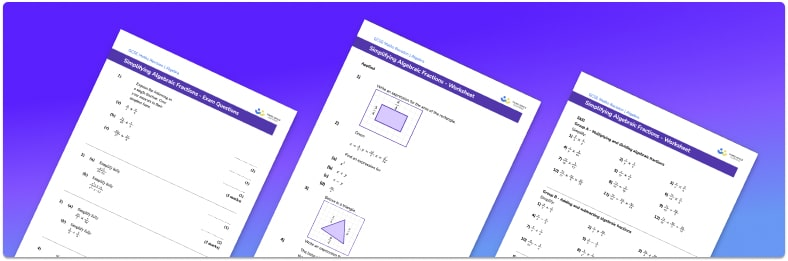• Section 1 of the simplifying algebraic fractions worksheet contains 20+ skills-based keyword questions, in 3 groups to support differentiation
• Section 2 contains 3 applied simplifying algebraic fractions questions with a mix of worded problems and deeper problem solving questions
• Section 3 contains 3 foundation and higher level GCSE exam style simplifying algebraic fractions questions
• Answers and a mark scheme for all simplifying algebraic fractions questions are provided
• Questions follow variation theory with plenty of opportunities for students to work independently at their own level
• All questions created by fully qualified expert secondary maths teachers

Suitable for GCSE maths revision for AQA, OCR and Edexcel exam boards

• This field is for validation purposes and should be left unchanged.

You can unsubscribe at any time (each email we send will contain an easy way to unsubscribe). To find out more about how we use your data, see our privacy policy.

### Simplifying algebraic fractions at a glance

Algebraic fractions are fractions where the numerator, denominator, or both are algebraic expressions. Algebraic fractions can be simplified by finding common factors between the numerator and denominator and cancelling them. These common factors could be integers or algebraic expressions.  Algebraic fractions are in their simplest form when there are no more common factors between the numerator and denominator.

Often we will need to factorise the numerator and/or denominator in order to identify common factors. Algebraic fractions can consist of linear expressions, quadratic expressions or other expressions involving larger indices.

As well as simplifying single algebraic fractions, we can simplify expressions involving adding/subtracting/multiplying/dividing algebraic fractions. The same rules apply here as with numeric fractions. For example, to add or subtract algebraic fractions, we first need to write the fractions with a common denominator. We can then add/subtract the numerators giving a single fraction.

Being able to work confidently with algebraic fractions is a skill that will come in useful for A Level maths.

Looking forward, students can then progress to additional simplifying expressions worksheets and other algebra worksheets, for example a sequences worksheet, simultaneous equations worksheet or straight line graphs worksheet.For more teaching and learning support on Algebra our GCSE maths lessons provide step by step support for all GCSE maths concepts.

## Related worksheets

Substitution Worksheet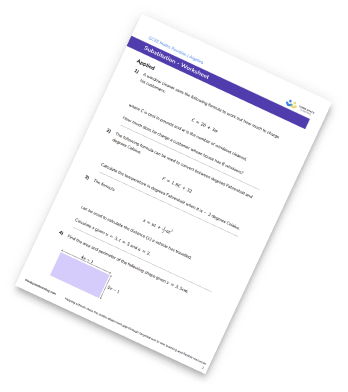Sketching Graphs Worksheet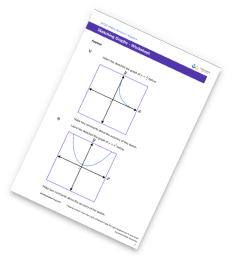Forming And Solving Equations Worksheet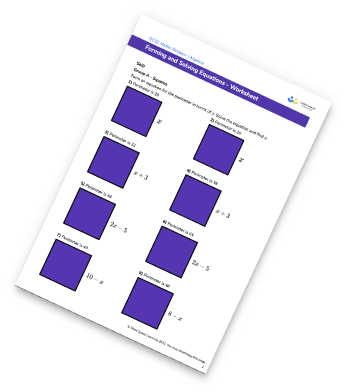Inverse Functions Worksheet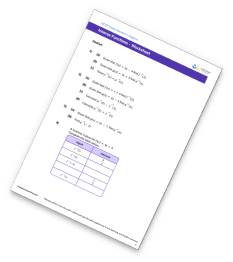## Do you have KS4 students who need more focused attention to succeed at GCSE?There will be students in your class who require individual attention to help them succeed in their maths GCSEs. In a class of 30, it’s not always easy to provide.

Help your students feel confident with exam-style questions and the strategies they’ll need to answer them correctly with our dedicated GCSE maths revision programme.

Lessons are selected to provide support where each student needs it most, and specially-trained GCSE maths tutors adapt the pitch and pace of each lesson. This ensures a personalised revision programme that raises grades and boosts confidence.

Find out more